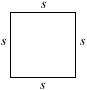index: click on a letter A B C D E F G H I J K L M N O P Q R S T U V W X Y Z A to Z index index: subject areas numbers & symbols sets, logic, proofs geometry algebra trigonometry advanced algebra & pre-calculus calculus advanced topics probability & statistics real world applications multimedia entrieswww.mathwords.com about mathwords website feedback

Square

A rectangle with all four sides of equal length. Formally, a square is a quadrilateral with four congruent sides and four congruent angles (all 90°).

 Square Area = s2